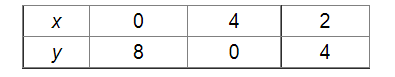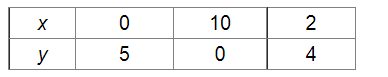# Show that the solution set of the following system of linear inequalities is an unbounded region:

Question:

Show that the solution set of the following system of linear inequalities is an unbounded region: $2 x+y \geq 8, x+2 y \geq 10, x \geq 0, y \geq 0$.

Solution:

We have:

$2 x+y \geq 8$           ...(i)

$x+2 y \geq 10$         ...(ii)

$x \geq 0$                  ....(iii)

$y \geq 0$                   ...(iv)

As, the solutions of the equation 2x + y = 8 are:As, the solutions of the equation x + 2y = 10 are:Now, the graph represented by the inequalities (i), (ii), (iii) and (iv) is as follows:Since, the common shaded region is the solution set of the given set of inequalities.

So, the solution set of the given linear inequalities is an unbounded region.